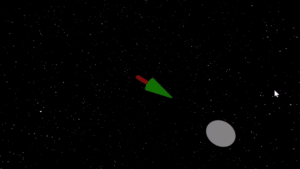# Smooth rotation

## Problem

You want to smoothly rotate a 3D object to point in a new direction.

## Solution

When you first encounter this problem, you may find yourself thinking in terms of Euler angles - the three values representing the angles to the x/y/z axes. While Godot will allow you to see the object’s Euler angles in the `rotation` property, it is not recommended to use them to work in 3D. There are a number of reasons why this the case, such as a problem called “gimbal lock”, where you lose one degree of freedom when one of your rotations reaches 90 degrees.

We can avoid using 3D Euler angles in Godot by using the object’s `Transform` property. This property represents the body’s position and orientation in space. It uses a mathematical construct called a matrix to do this, but you don’t really need to understand the underlying math in order to make use of it.

### `look_at()`

Let’s say we have a 3D object such as a missile or arrow and you want it to point at its target. Wecan do this using the `Spatial` method `look_at()`:

``````func _process(delta):
var target_position = \$Target.transform.origin
\$Arrow.look_at(target_position, Vector3.UP)
``````

This code would make our node (`\$Arrow`) always point at the target’s position, no matter how it moves.Note that `look_at()` requires 2 parameters: the target position, and an “up vector”. Imagine an airplane pointing its nose towards a target - there are an infinite number of ways it could be oriented, because the plane could roll about its axis. This second parameter is how you define what you want the final orientation to be.

### Smooth rotation

The above code works, but it snaps the rotation instantly to the target. This might be fine if you have a very slow-moving target, but looks unnatural. It would look better if we move smoothly, or “interpolated”, the rotation smoothly between the starting orientation and the ending.

Godot has us covered here too, because the `Transform` object has a method called `interpolate_with()`, which returns an intermediate transform between a current one and a target one.

``````var speed = 5

func _process(delta):
var target_position = \$Target.transform.origin
var new_transform = \$Arrow.transform.looking_at(target_position, Vector3.UP)
\$Arrow.transform  = \$Arrow.transform.interpolate_with(new_transform, speed * delta)
``````Note that since `interpolate_with()` operates on the `transform`, it can be used to interpolate both rotation and position of an object.

## Wrapping up

That’s it! Use this handy method to rotate your 3D objects, and stop thinking about angles!